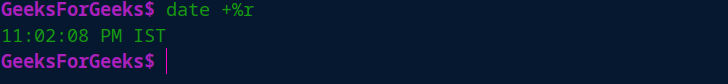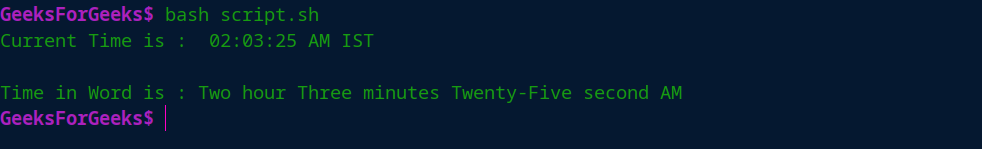# Shell Script to Display Time in Word

• Last Updated : 02 Nov, 2021

Linux/Unix has the inbuilt functionality to show the current time. Usually, Linux shows the current time in general format rather than word, and with this may some user can disagree.

So, To show the current time in a better and pretty format here is given a bash script. It shows the current time in 12-hour format that will be in word style rather than general.

If we direct run the time command in Linux or Unix then will get output like following

```Command: date +%r
Output: 11:02:08 PM IST```Our task is to Display Time in Word:

Example:

```Current Time is :  02:03:25 AM IST

Time in Word is : Two hour Three minutes Twenty-Five second AM```

Script Code Approach: To write the script for display the time in the world, we require some simple Linux inbuilt commands like “date”, “echo” ,

1. Some basic shell scripting and through this, follow the below steps to write the script code.
2. Get all time components like hour, minutes, and seconds in different variables.
3. Make an array of strings that has the number from 0 to 19 in a word and use this word using the array index.
4. Make another array of strings that will contain the value like 0,10,20,30,40,50,60 in word.
5. Now, change the 24-hour format to a 12-hour format using an if-else statement.
6. Create a function that takes an integer argument and returns that integer in word ( here number can be up to 2 digits because in time there is only number up to 2 digits).
7. Now, convert all the components of time in the word and print the whole string of time using the “echo” command.

Script Code:

```# ## bash script to display Time in word

# print current time in original function format of Linux/Unix
echo "Current Time is : " `date +%r`
echo

# getting hour, minutes and seconds value in separate as integer
hour=`date +%-H`
minutes=`date +%-M`
seconds=`date +%-S`
post='AM'

# create array of strings two show time in word
time=(Zero One Two Three Four Five Six Seven Eight Nine
Ten Eleven Twelve Thirteen Fourteen Fifteen
Sixteen Seventeen Eighteen Nineteen)

time_ten=(Zero Ten Twenty Thirty Forty Fifty Sixty)

# check hour is greater than "12" or not
# if it than use 12-hour format to show the current time
if [[ \$hour -gt 11 ]]
then
let "hour -= 12"
post="PM"
fi

# check if hour is 00 then print 12AM
if [[ \$hour -eq 0 ]]
then
let "hour = 12"
fi

# Function for get current hour,minutes and seconds in words
function getNumber(){

# check condition when time is less than 20 and get value directly from array
if [[ \$1 -lt 20 ]]
then
timeInWord=\${time[\$1]}
else
# else block when time is greater than 20 ( minutes and seconds can be up to 59)
f=`expr \$1 / 10`
s=`expr \$1 % 10`

# check condition when first part of the time is zero than we don't have to print that value
if [[ \$s -eq 0 ]]
then
timeInWord=\${time_ten[\$f]}
else
timeInWord="\${time_ten[\$f]}-\${time[\$s]}"
fi
fi
}

# make gloabal variable for timeInWord
timeInWord = "Geeks For Geeks"

# get hour in word
getNumber \$hour
hourInWord=\$timeInWord

# get minutes in word
getNumber \$minutes
minutesInWord=\$timeInWord

# get seconds in word
getNumber \$seconds
secondsInWord=\$timeInWord

# print time in usual and simple word format in one line
echo "Time in Word is : \$hourInWord hour \$minutesInWord minutes \$secondsInWord second \$post"

# END```

Output:My Personal Notes arrow_drop_up